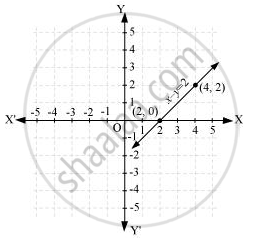# Draw the graph of the following linear equations in two variables:- x – y = 2 - Mathematics

Draw the graph of the following linear equations in two variables:- x – y = 2

#### Solution

It can be observed that x = 4, y = 2 and x = 2, y = 0 are solutions of the above equation. Therefore, the solution table is as follows.

 x 4 2 y 2 0

The graph of the above equation is constructed as follows.Concept: Graph of a Linear Equation in Two Variables
Is there an error in this question or solution?

#### APPEARS IN

NCERT Class 9 Maths
Chapter 4 Linear Equations in two Variables
Exercise 4.3 | Q 1.2 | Page 74
RD Sharma Mathematics for Class 9
Chapter 7 Linear Equations in Two Variables
Exercise 7.3 | Q 1.2

Share